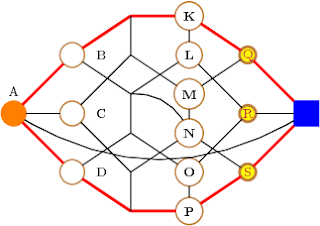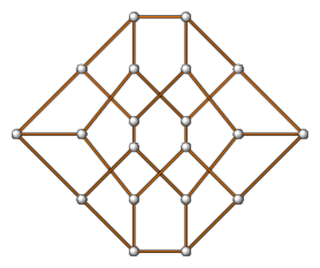## Friday, February 26, 2010

### This blog moves

This blog will continue here, for reasons explained there.

## Saturday, September 05, 2009

### A 3d graph... after the example in the tikz gallery.

\usetikzlibrary{calc,3d}
\newcommand{\setxyz}{%
\pgfmathsetmacro{\xone}{cos(180+#1)}%
\pgfmathsetmacro{\yone}{sin(180+#1)}%
\pgfmathsetmacro{\xtwo}{cos(360-#1)}%
\pgfmathsetmacro{\ytwo}{sin(360-#1)}%
}
\setxyz{17}
\begin{tikzpicture}%
[x = {(\xone cm,\yone cm)},
y = {(\xtwo cm,\ytwo cm)},
z = {(0cm,1cm)}]
\SetVertexNoLabel
\begin{scope}[canvas is xy plane at z=-5]
\Vertex{x}
\end{scope}
\begin{scope}[canvas is xy plane at z=0]
\grEmptyCycle[prefix=a]{5}
\end{scope}
\EdgeFromOneToAll{x}{a}{}{5}
\Edges(a0,a1,a2,a3,a4,a0)
\begin{scope}[canvas is xy plane at z=5]
\Vertex{y}
\end{scope}
\EdgeFromOneToAll{y}{a}{}{5}
\end{tikzpicture}

## Friday, August 28, 2009

### A circulant tournamentThis is the circulant C_9(1,2,3,4)

\begin{tikzpicture}
\usepgflibrary{arrows}
\GraphInit[vstyle=Art]
\SetUpEdge[style={->,>=angle 45,bend right=10},color=red]
\grCirculant[RA=3]{9}{1,-2,3,-4}
\end{tikzpicture}

## Tuesday, August 18, 2009

### Several options for the \Vertex macro

UPDATE: This example no longer works with the latest versions of tkz-graph and tkz-berge. For an updated version see this post at my new blog.\begin{tikzpicture}
\SetVertexNormal[LineColor=brown]
\Vertex[x=0,y=2.5,style=orange,LabelOut=true,Lpos=90]{A}
{\tikzstyle{every node} = [node distance=1.5cm]
\Vertices[x=1.5,y=4,dir=\SO,LabelOut=true,Ldist=5pt]{B,C,D}}
\Vertices[x=3,y=5,dir=\SO,style={shape=coordinate}]{E,F,G,H,I,J}
\Vertices[x=4.5,y=5,dir=\SO,style={font=\bfseries}]{K,L,M,N,O,P}
{\tikzstyle{every node} = [node distance=1.5cm]
\Vertices[x=6,y=4,dir=\SO,
style={line width=2pt,
inner sep=0pt,
text=purple,
fill=yellow,
minimum size=12pt}]{Q,R,S}}
\Vertex[x=7.5,y=2.5,style={shape=rectangle,blue}]{T}
\Edges[color=red,lw=2pt](A,B,E,K,Q,T,S,P,J,D,A)
\Edges(B,G,L,R,T)
\Edges(D,H,O,R)
\Edges(A,C,F,M,Q)
\Edges(C,I,N,S)
\foreach \x/\y in {E/F,G/H,I/J,K/L,M/N,O/P}
{\Edge(\x)(\y)}
\Edge[style={bend left}](G)(N)
\Edge[style={bend right}](A)(T)
\end{tikzpicture}

## Monday, August 03, 2009

### Several options for the \Edge macro\begin{tikzpicture}
\SetVertexNormal
\Vertex[x=0,y=2.5]{A}
{\tikzstyle{every node} = [node distance=1.5cm]
\Vertices[x=1.5,y=4,dir=\SO]{B,C,D}}
\Vertices[x=3,y=5,dir=\SO]{E,F,G,H,I,J}
\Vertices[x=4.5,y=5,dir=\SO]{K,L,M,N,O,P}
{\tikzstyle{every node} = [node distance=1.5cm]
\Vertices[x=6,y=4,dir=\SO]{Q,R,S}}
\Vertex[x=7.5,y=2.5]{T}
\Edges[color=red](A,B,E,K,Q,T,S,P,J,D,A)
\foreach \x/\y in {E/F,G/H,I/J,K/L,M/N,O/P}
{\Edge[color=green](\x)(\y)}
\Edge[style=->](I)(P)
\Edge[style=dashed](A)(C)
\Edge[style=dotted](C)(F)
\Edge[label=CD](C)(D)
\Edge[label=BC,labelcolor=yellow](B)(C)
\Edge[label=QR,labelcolor=orange,labeltext=cyan](Q)(R)
\Edge[label=RS,labelstyle=right](R)(S)
\Edge[label=OS,labelstyle={below=1pt,inner sep=0pt}](O)(S)
\Edge[label=LQ,labelstyle={above,font=\bfseries}](L)(Q)
\Edge[label=FL,labelstyle={font=\tiny\bfseries}](F)(L)
\Edge[label=GN,labelstyle={sloped,above}](G)(N)
\Edge[style={bend right}](A)(T)
\end{tikzpicture}

### Simulating KTikZ with Emacs

I stumbled upon a piece of software called KtikZ. The screenshot in that page, together with the fact that Doc-View mode is included in the new Emacs 23, inspired me to try that idea with tikz2pdf. With help from folks from gnu.emacs.help, I came up with the following function:

(defun split-for-tikz2pdf ()
(interactive)
(TeX-run-style-hooks "tikz")
(TeX-run-style-hooks "tkz-berge")
(split-window-horizontally 60)
(other-window 1)
(split-window-vertically 15)
(find-file "tikz2pdf_temp.pdf")
(doc-view-mode)
(auto-revert-mode 1)
(other-window 1)
(let ((file-path (buffer-file-name)))
(when file-path
(shell)
(comint-send-string (get-buffer-process (current-buffer))
(format "tikz2pdf -v %S \n" file-path))))
)

which gives something like this, when applied at a buffer with a .tex file:Unfortunately, the buffer for the .pdf file is not in Doc-View mode after executing the function. One has to go to that window and do M-x doc-view-mode. After that, sometimes the picture is not generated correctly and one has to reload manually with g. Other useful Doc-View key bindings are + and -.

You might want to use my simple AucTeX style files tikz.el and tkz-berge.el.

## Thursday, February 21, 2008

### Using tikz2pdf

When drawing graphs by trial and error, the script tikz2pdf by Hans Meine can be very useful.

I have the following in ~/.tikz2pdf.tex:

\documentclass{article}

\usepackage{tikz}
\usepackage{tkz-berge}

\usepackage[graphics,tightpage,active]{preview}
\PreviewEnvironment{tikzpicture}
\newlength{\imagewidth}
\newlength{\imagescale}

\begin{document}

\input{%s}

\end{document}

Then, I edit a file, say foo.tikz, with

\begin{tikzpicture}
\grHeawood[RA=1]
\end{tikzpicture}

and run

tikz2pdf -v foo.tikz

I can open then the generated tikz2pdf_temp.pdf with, say Sumatra,which is a viewer that, unlike Acrobat, does not complain if an opened file is modified. Next time I modify foo.tikz and save it, pdflatex is automatically run and I can press r in Sumatra to update the picture.

## Thursday, May 10, 2007

### New LaTeX package for graphsAlain Matthes has produced a package (tkz-berge.sty) which should become THE way to draw graphs in LaTeX. It is built on top of Tikz, and so can be easily integrated with beamer presentations. The package can be dowloaded from here, and the documentation (in French, but very nicely formatted and with lot of examples) can be obtained from here. Alain's packages are all here.

I plan to redraw all my examples using tkz-berge's syntax, but for the time being, I will show code for the dodecahedron above:

\documentclass{article}

\usepackage{tikz}
\usepackage{tkz-berge}

\pagestyle{empty}

\tikzstyle{NormalVertexStyle}=[shape = circle,%
ball color = white,%
very thin,
inner sep=3pt,%
draw]
\SetVertexNoLabel
\tikzstyle{EdgeStyle}= [thick,%
double= orange,%
double distance = 1pt]

\begin{document}
\begin{center}
\begin{tikzpicture}
\tikzstyle{every node} = [node distance=1.5cm]
\Vertex(A)
\EA{A}(C) \NO{C}(B) \SO{C}(D)
\tikzstyle{every node} = [node distance=1.2cm]
\EA{B}(F) \NO{F}(E) \SO{F}(G)
\EA{D}(I) \NO{I}(H) \SO{I}(J)
\EA{F}(L) \NO{L}(K) \SO{L}(M)
\EA{I}(O) \NO{O}(N) \SO{O}(P)
\EA{L}(Q)
\tikzstyle{every node} = [node distance=1.5cm]
\SO{Q}(R) \SO{R}(S) \EA{R}(T)
\Edge(A)(B) \Edge(A)(C) \Edge(A)(D)
\Edge(B)(E) \Edge(B)(G)
\Edge(C)(F) \Edge(C)(I)
\Edge(D)(H) \Edge(D)(J)
\Edge(E)(K) \Edge(E)(F) \Edge(F)(M)
\Edge(G)(L) \Edge(G)(H) \Edge(H)(O)
\Edge(I)(N) \Edge(I)(J) \Edge(J)(P)
\Edge(K)(Q) \Edge(K)(L)
\Edge(M)(Q) \Edge(M)(N)
\Edge(T)(Q) \Edge(L)(R)
\Edge(O)(R) \Edge(O)(P)
\Edge(T)(R) \Edge(N)(S)
\Edge(P)(S) \Edge(T)(S)
\end{tikzpicture}
\end{center}
\end{document}